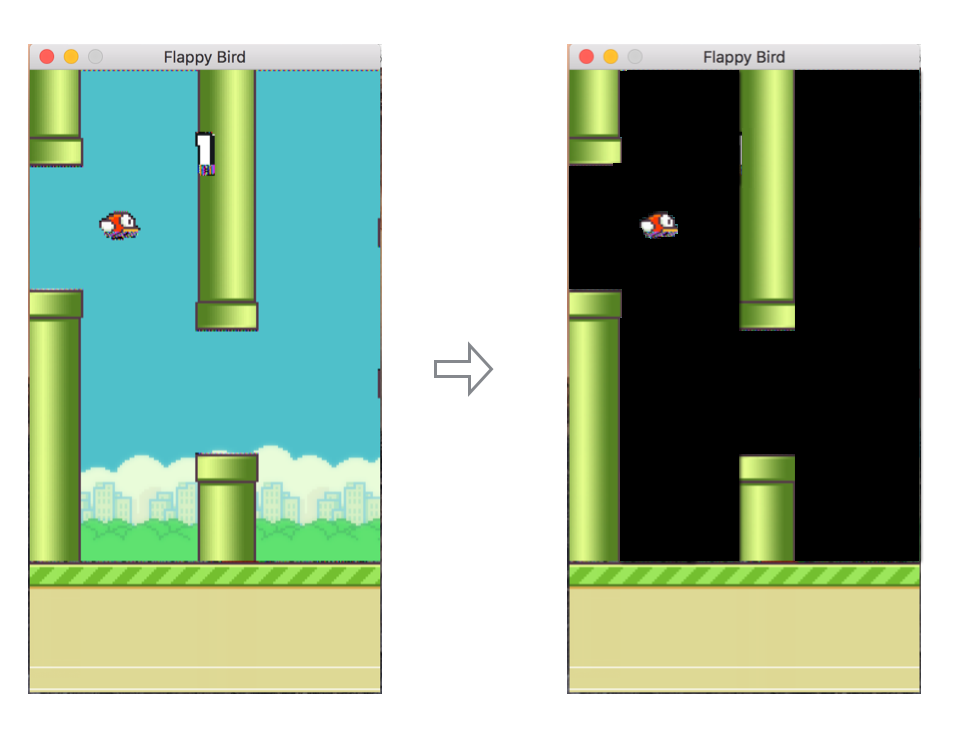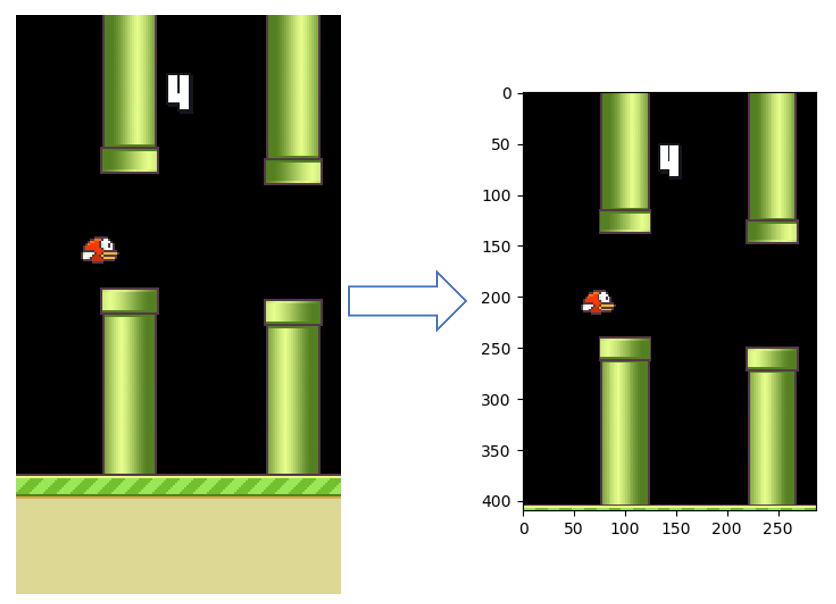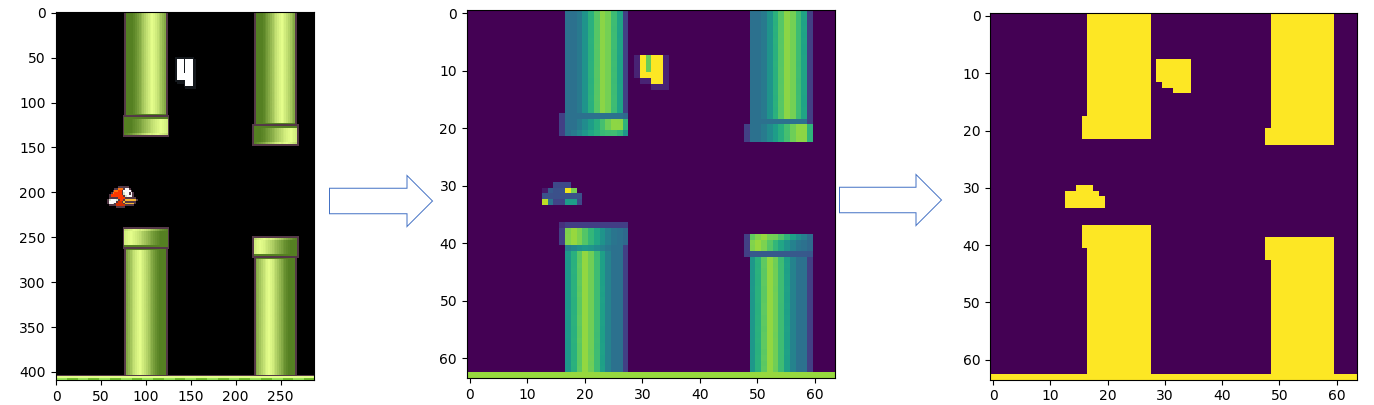# Deep Q Network

An implementation of q algorithm of Reinforcement Learning.

## Installation Dependencies:

1. Python 3
2. TensorFlow 1.0.1
3. pygame
4. gym

## How to Run?

``````git clone https://github.com/lufficc/dqn.git
cd dqn
python run.py
``````

## Tricks for flappybird

Remove background image:clip useless part:resize and using binary image:decayed ε-greedy exploration, and when exploration, 0.95 probability to do nothing(because in flappy bird, most time wo do nothing). This is very important. It makes model converge in less than 2 hours.

``````def egreedy_action(self, state):
#Exploration
if random.random() <= self.epsilon:
if random.random() < 0.95:
action_index = 0
else:
action_index = 1
# action_index = random.randint(0, self.num_actions - 1)
else:
#Exploitation
action_index = self.action(state)
if self.epsilon > self.final_epsilon:
self.epsilon *= self.decay_factor
return action_index
``````

## Thanks

Get A Weekly Email With Trending Projects For These Topics
No Spam. Unsubscribe easily at any time.
Python (1,123,251
Machine Learning (29,964
Reinforcement Learning (3,769
Q Learning (472
Related Projects